# Straight Lines ( Especially For XI )

27 de Dec de 2014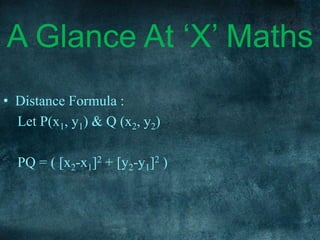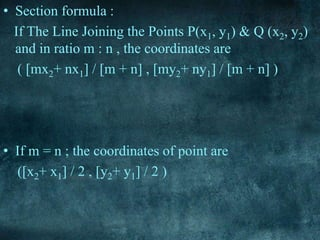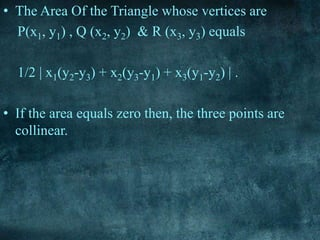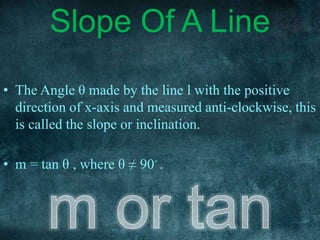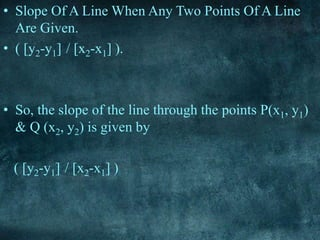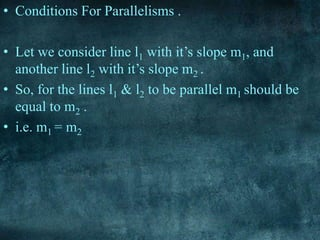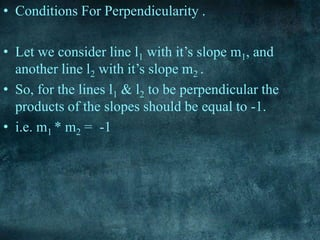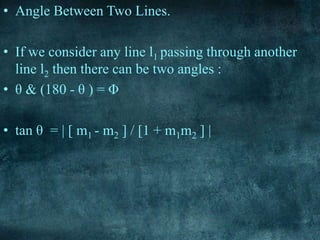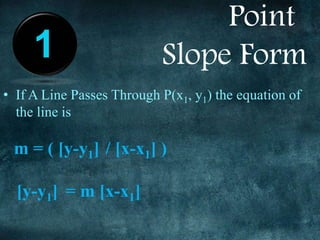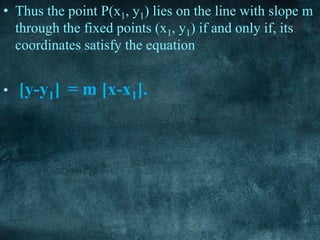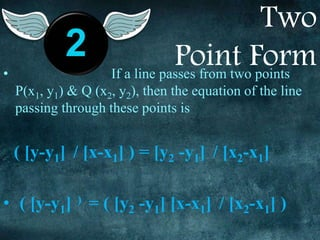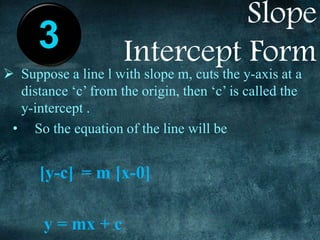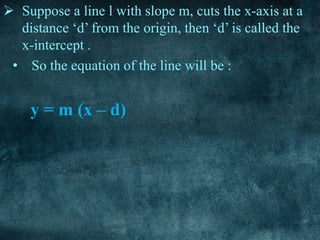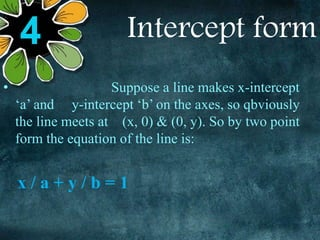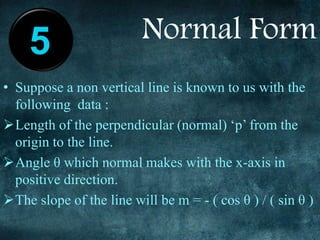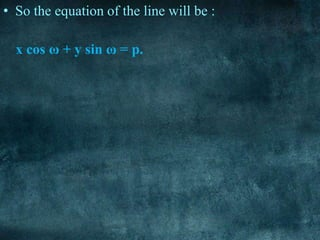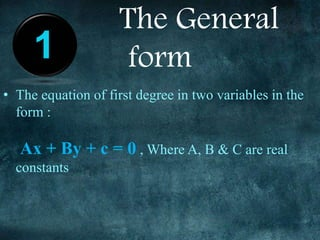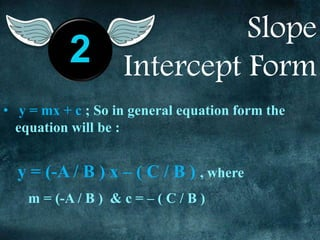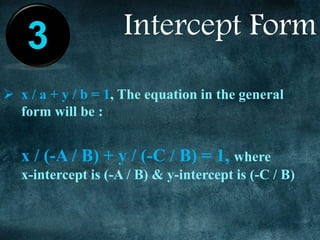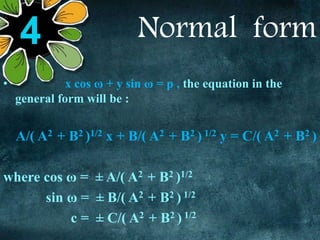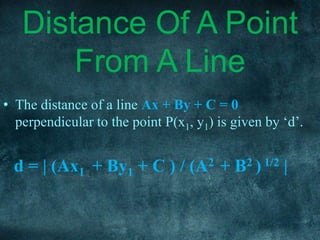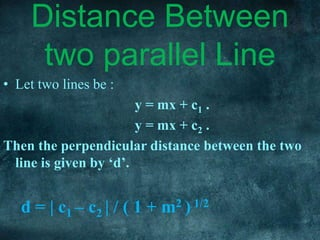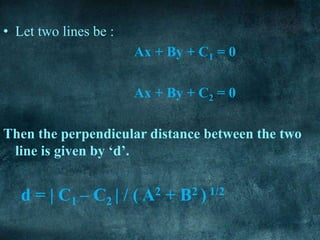1 de 33

### Straight Lines ( Especially For XI )

• 3. Topic : Straight Line By: Atit S Gaonkar
• 6. EQUATIONS A GLANCE AT X MATHS A LINESLOPE OF INDEXA
• 7. A Glance At ‘X’ Maths • Distance Formula : Let P(x1, y1) & Q (x2, y2) PQ = ( [x2-x1]2 + [y2-y1]2 )
• 8. • Section formula : If The Line Joining the Points P(x1, y1) & Q (x2, y2) and in ratio m : n , the coordinates are ( [mx2+ nx1] / [m + n] , [my2+ ny1] / [m + n] ) • If m = n ; the coordinates of point are ([x2+ x1] / 2 , [y2+ y1] / 2 )
• 9. • The Area Of the Triangle whose vertices are P(x1, y1) , Q (x2, y2) & R (x3, y3) equals 1/2 | x1(y2-y3) + x2(y3-y1) + x3(y1-y2) | . • If the area equals zero then, the three points are collinear.
• 10. • The Angle θ made by the line l with the positive direction of x-axis and measured anti-clockwise, this is called the slope or inclination. • m = tan θ , where θ ≠ 90◦ . Slope Of A Line
• 11. • Slope Of A Line When Any Two Points Of A Line Are Given. • ( [y2-y1] / [x2-x1] ). • So, the slope of the line through the points P(x1, y1) & Q (x2, y2) is given by ( [y2-y1] / [x2-x1] )
• 12. • Conditions For Parallelisms . • Let we consider line l1 with it’s slope m1, and another line l2 with it’s slope m2 . • So, for the lines l1 & l2 to be parallel m1 should be equal to m2 . • i.e. m1 = m2
• 13. • Conditions For Perpendicularity . • Let we consider line l1 with it’s slope m1, and another line l2 with it’s slope m2 . • So, for the lines l1 & l2 to be perpendicular the products of the slopes should be equal to -1. • i.e. m1 * m2 = -1
• 14. • Angle Between Two Lines. • If we consider any line l1 passing through another line l2 then there can be two angles : • θ & (180 - θ ) = Φ • tan θ = | [ m1 - m2 ] / [1 + m1m2 ] |
• 17. • If A Line Passes Through P(x1, y1) the equation of the line is m = ( [y-y1] / [x-x1] ) [y-y1] = m [x-x1] 1 Point Slope Form
• 18. • Thus the point P(x1, y1) lies on the line with slope m through the fixed points (x1, y1) if and only if, its coordinates satisfy the equation • [y-y1] = m [x-x1].
• 19. Two Point Form• If a line passes from two points P(x1, y1) & Q (x2, y2), then the equation of the line passing through these points is ( [y-y1] / [x-x1] ) = [y2 -y1] / [x2-x1] • ( [y-y1] ) = ( [y2 -y1] [x-x1] / [x2-x1] ) 2
• 20. Slope Intercept Form Suppose a line l with slope m, cuts the y-axis at a distance ‘c’ from the origin, then ‘c’ is called the y-intercept . • So the equation of the line will be [y-c] = m [x-0] y = mx + c 3
• 21.  Suppose a line l with slope m, cuts the x-axis at a distance ‘d’ from the origin, then ‘d’ is called the x-intercept . • So the equation of the line will be : y = m (x – d)
• 22. Intercept form • Suppose a line makes x-intercept ‘a’ and y-intercept ‘b’ on the axes, so qbviously the line meets at (x, 0) & (0, y). So by two point form the equation of the line is: x / a + y / b = 1 4
• 23. Normal Form • Suppose a non vertical line is known to us with the following data : Length of the perpendicular (normal) ‘p’ from the origin to the line. Angle θ which normal makes with the x-axis in positive direction. The slope of the line will be m = - ( cos θ ) / ( sin θ ) 5
• 24. • So the equation of the line will be : x cos ω + y sin ω = p.
• 26. • The equation of first degree in two variables in the form : Ax + By + c = 0 , Where A, B & C are real constants 1 The General form
• 27. Slope Intercept Form • y = mx + c ; So in general equation form the equation will be : y = (-A / B ) x – ( C / B ) , where m = (-A / B ) & c = – ( C / B ) 2
• 28. Intercept Form  x / a + y / b = 1, The equation in the general form will be : x / (-A / B) + y / (-C / B) = 1, where x-intercept is (-A / B) & y-intercept is (-C / B) 3
• 29. Normal form • x cos ω + y sin ω = p , the equation in the general form will be : A/( A2 + B2 )1/2 x + B/( A2 + B2 ) 1/2 y = C/( A2 + B2 ) where cos ω = ± A/( A2 + B2 )1/2 sin ω = ± B/( A2 + B2 ) 1/2 c = ± C/( A2 + B2 ) 1/2 4
• 30. • The distance of a line Ax + By + C = 0 perpendicular to the point P(x1, y1) is given by ‘d’. d = | (Ax1 + By1 + C ) / (A2 + B2 ) 1/2 | Distance Of A Point From A Line
• 31. • Let two lines be : y = mx + c1 . y = mx + c2 . Then the perpendicular distance between the two line is given by ‘d’. d = | c1 – c2 | / ( 1 + m2 ) 1/2 Distance Between two parallel Line
• 32. • Let two lines be : Ax + By + C1 = 0 Ax + By + C2 = 0 Then the perpendicular distance between the two line is given by ‘d’. d = | C1 – C2 | / ( A2 + B2 ) 1/2

### Notas do Editor

1. Presentations are a powerful communication medium.
2. Presentations are a powerful communication medium.
3. Presentations are a powerful communication medium.
4. …global causes.
5. Along the way we’ve discovered…
6. …five simple rules for creating world-changing presentations.
7. …is destructive.